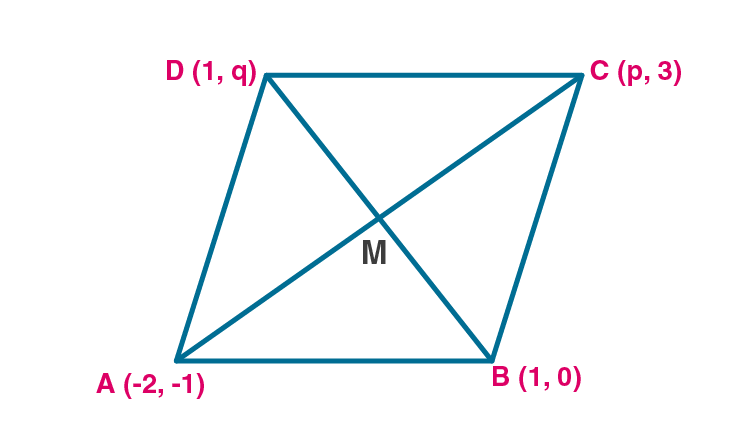Newbie

# If the points A ( – 2, – 1), B (1, 0), C (p, 3) and D (1, q) form a parallelogram ABCD, find the values of p and q.

• 2

An Important Question of M.L Aggarwal book of class 10 Based on Section Formula Chapter for ICSE BOARD.
If four points form a parallelogram then find the value aksed in the question inside that point.
This is the Question Number 24, Exercise 11 of M.L Aggarwal.

Share

1.Given vertices of the parallelogram are A(-2,-1), B(1, 0), C(p,3) and D(1,q).

Let M(x,y) be the midpoint of the diagonals of the parallelogram ABCD.

Diagonals AC and BD bisect each other at M.

When M is the midpoint of AC

By midpoint formula,

x = (-2+p)/2 = (p-2)/2 ..(i)

y = (-1+3)/2 = 2/2 = 1 ..(ii)

When M is the midpoint of BD

By midpoint formula,

x = (1+1)/2 = 2/2 = 1 ..(iii)

y = (q+0)/2 = q/2 ..(iv)

Equating (i) and (iii), we get

(p-2)/2 = 1

p-2 = 2

p = 2+2 = 4

Equating (i2) and (iv), we get

q/2 = 1

q = 2

Hence the value of p and q are 4 and 2 respectively.

• 1